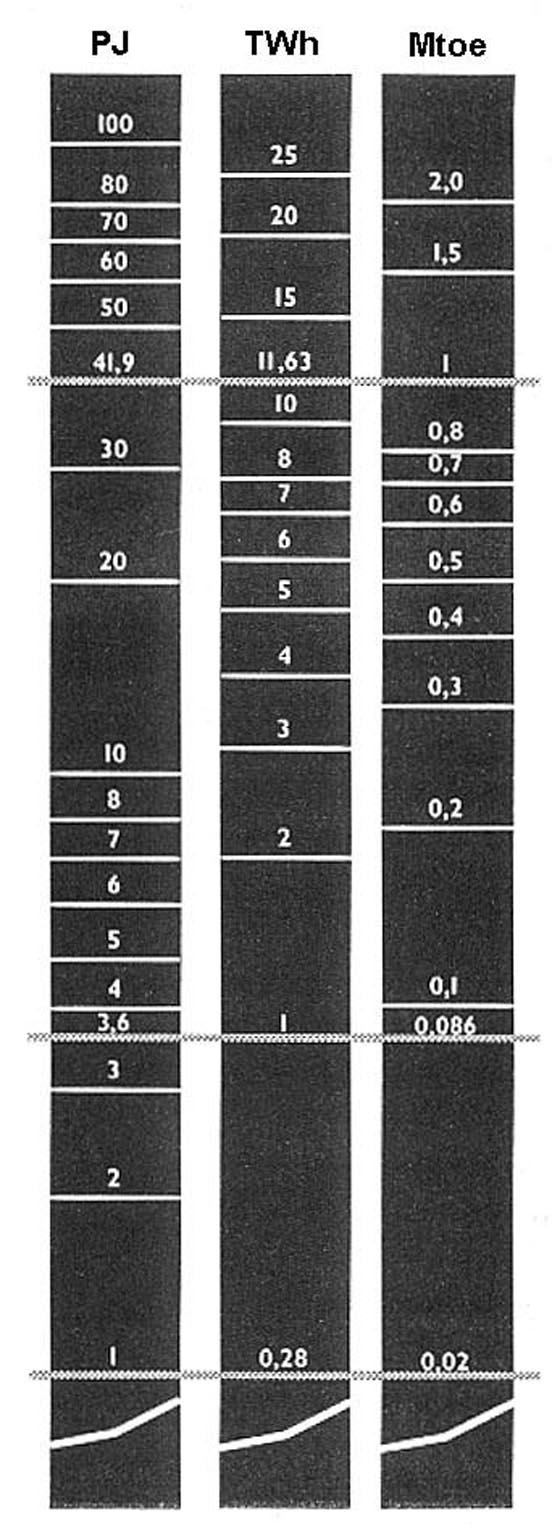# Единицы измерений

## 1. Энергетические единицы

1 J (joule) = 1 Ws = 4.1868 cal

1 GJ (gigajoule) = 10 E9 J

1 TJ (terajoule) = 10 E12 J

1 PJ (petajoule) = 10 E15 J

1 (kilowatt hour) kWh = 3,600,000 Joule

1 toe (tonne oil equivalent)

= 7.4 barrels of crude oil in primary energy

= 7.8 barrels in total final consumption

= 1270 m3 of natural gas

= 2.3 metric tonnes of coal *)

1 Mtoe (million tonne oil equivalent) = 41.868 PJ

## 2. Электроэнергия

Electrical power is usually measured in watt (W), kilowatt (kW), megawatt (MW), etc.

Power is energy transfer per unit of time.

1 kW =1000 W

1 MW = 1 000 000 W

1 GW = 1 000 MW

1 TW = 1 000 000 MW

Power (e.g. in W) may be measured at any point in time, whereas energy (e.g. in kWh) has to be measured during a certain period, e.g. a second, an hour, or a year.

## 3. Аббревиатуры

m = metre = 3.28 ft.

s = second

h = hour

W = Watt

HP = horsepower

J = Joule

cal = calorie

toe = tonnes of oil equivalent

Hz= Hertz (cycles per second)

10 E-12 = p pico = 1/1000,000,000,000

10 E-9 = n nano = 1/1000,000,000

10 E-6 = µ micro = 1/1000,000

10 E-3 = m mili = 1/1000

10 E3 = k kilo = 1,000 = thousands

10 E6 = M mega = 1,000,000 = millions

10 E9 = G giga = 1,000,000,000

10 E12 = T tera = 1,000,000,000,000

10 E15 = P peta = 1,000,000,000,000,000

## 4. Скорость ветра

1 m/s = 3.6 km/h = 2.187 mph = 1.944 knots

1 knot = 1 nautical mile per hour = 0.5144 m/s = 1.852 km/h = 1.125 mph

## 5. PJ, TWh, Mtoe